# Tag: necklace

It’s been a while, so let’s include a recap : a (transitive) permutation representation of the modular group $\Gamma = PSL_2(\mathbb{Z})$ is determined by the conjugacy class of a cofinite subgroup $\Lambda \subset \Gamma$, or equivalently, to a dessin d’enfant. We have introduced a quiver (aka an oriented graph) which comes from a triangulation of the compactification of $\mathbb{H} / \Lambda$ where $\mathbb{H}$ is the hyperbolic upper half-plane. This quiver is independent of the chosen embedding of the dessin in the Dedeking tessellation. (For more on these terms and constructions, please consult the series Modular subgroups and Dessins d’enfants).

Why are quivers useful? To start, any quiver $Q$ defines a noncommutative algebra, the path algebra $\mathbb{C} Q$, which has as a $\mathbb{C}$-basis all oriented paths in the quiver and multiplication is induced by concatenation of paths (when possible, or zero otherwise). Usually, it is quite hard to make actual computations in noncommutative algebras, but in the case of path algebras you can just see what happens.

Moreover, we can also see the finite dimensional representations of this algebra $\mathbb{C} Q$. Up to isomorphism they are all of the following form : at each vertex $v_i$ of the quiver one places a finite dimensional vectorspace $\mathbb{C}^{d_i}$ and any arrow in the quiver
$$\xymatrix{\vtx{v_i} \ar[r]^a & \vtx{v_j}}$$ determines a linear map between these vertex spaces, that is, to $a$ corresponds a matrix in $M_{d_j \times d_i}(\mathbb{C})$. These matrices determine how the paths of length one act on the representation, longer paths act via multiplcation of matrices along the oriented path.

A necklace in the quiver is a closed oriented path in the quiver up to cyclic permutation of the arrows making up the cycle. That is, we are free to choose the start (and end) point of the cycle. For example, in the one-cycle quiver

$$\xymatrix{\vtx{} \ar[rr]^a & & \vtx{} \ar[ld]^b \\ & \vtx{} \ar[lu]^c &}$$

the basic necklace can be represented as $abc$ or $bca$ or $cab$. How does a necklace act on a representation? Well, the matrix-multiplication of the matrices corresponding to the arrows gives a square matrix in each of the vertices in the cycle. Though the dimensions of this matrix may vary from vertex to vertex, what does not change (and hence is a property of the necklace rather than of the particular choice of cycle) is the trace of this matrix. That is, necklaces give complex-valued functions on representations of $\mathbb{C} Q$ and by a result of Artin and Procesi there are enough of them to distinguish isoclasses of (semi)simple representations! That is, linear combinations a necklaces (aka super-potentials) can be viewed, after taking traces, as complex-valued functions on all representations (similar to character-functions).

In physics, one views these functions as potentials and it then interested in the points (representations) where this function is extremal (minimal) : the vacua. Clearly, this does not make much sense in the complex-case but is relevant when we look at the real-case (where we look at skew-Hermitian matrices rather than all matrices). A motivating example (the Yang-Mills potential) is given in Example 2.3.2 of Victor Ginzburg’s paper Calabi-Yau algebras.

Let $\Phi$ be a super-potential (again, a linear combination of necklaces) then our commutative intuition tells us that extrema correspond to zeroes of all partial differentials $\frac{\partial \Phi}{\partial a}$ where $a$ runs over all coordinates (in our case, the arrows of the quiver). One can make sense of differentials of necklaces (and super-potentials) as follows : the partial differential with respect to an arrow $a$ occurring in a term of $\Phi$ is defined to be the path in the quiver one obtains by removing all 1-occurrences of $a$ in the necklaces (defining $\Phi$) and rearranging terms to get a maximal broken necklace (using the cyclic property of necklaces). An example, for the cyclic quiver above let us take as super-potential $abcabc$ (2 cyclic turns), then for example

$\frac{\partial \Phi}{\partial b} = cabca+cabca = 2 cabca$

(the first term corresponds to the first occurrence of $b$, the second to the second). Okay, but then the vacua-representations will be the representations of the quotient-algebra (which I like to call the vacualgebra)

$\mathcal{U}(Q,\Phi) = \frac{\mathbb{C} Q}{(\partial \Phi/\partial a, \forall a)}$

which in ‘physical relevant settings’ (whatever that means…) turn out to be Calabi-Yau algebras.

But, let us return to the case of subgroups of the modular group and their quivers. Do we have a natural super-potential in this case? Well yes, the quiver encoded a triangulation of the compactification of $\mathbb{H}/\Lambda$ and if we choose an orientation it turns out that all ‘black’ triangles (with respect to the Dedekind tessellation) have their arrow-sides defining a necklace, whereas for the ‘white’ triangles the reverse orientation makes the arrow-sides into a necklace. Hence, it makes sense to look at the cubic superpotential $\Phi$ being the sum over all triangle-sides-necklaces with a +1-coefficient for the black triangles and a -1-coefficient for the white ones. Let’s consider an index three example from a previous post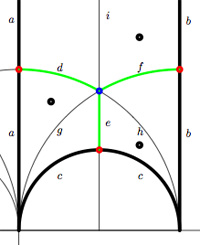$$\xymatrix{& & \rho \ar[lld]_d \ar[ld]^f \ar[rd]^e & \\ i \ar[rrd]_a & i+1 \ar[rd]^b & & \omega \ar[ld]^c \\ & & 0 \ar[uu]^h \ar@/^/[uu]^g \ar@/_/[uu]_i &}$$

In this case the super-potential coming from the triangulation is

$\Phi = -aid+agd-cge+che-bhf+bif$

and therefore we have a noncommutative algebra $\mathcal{U}(Q,\Phi)$ associated to this index 3 subgroup. Contrary to what I believed at the start of this series, the algebras one obtains in this way from dessins d’enfants are far from being Calabi-Yau (in whatever definition). For example, using a GAP-program written by Raf Bocklandt Ive checked that the growth rate of the above algebra is similar to that of $\mathbb{C}[x]$, so in this case $\mathcal{U}(Q,\Phi)$ can be viewed as a noncommutative curve (with singularities).

However, this is not the case for all such algebras. For example, the vacualgebra associated to the second index three subgroup (whose fundamental domain and quiver were depicted at the end of this post) has growth rate similar to that of $\mathbb{C} \langle x,y \rangle$…

I have an outlandish conjecture about the growth-behavior of all algebras $\mathcal{U}(Q,\Phi)$ coming from dessins d’enfants : the algebra sees what the monodromy representation of the dessin sees of the modular group (or of the third braid group).
I can make this more precise, but perhaps it is wiser to calculate one or two further examples…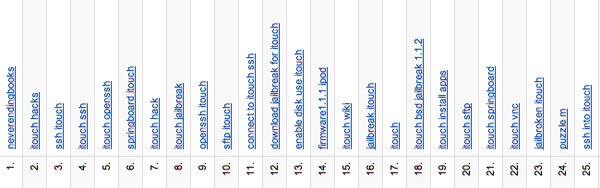Kea’s post reminded me to have a look at my search terms (the things people type into search engines to get redirected here). Quite a sobering experience…

Via Google Analytics I learn that 49,51% of traffic comes from Search Engines (compared to 26,17% from Referring Sites and 24,32% from direct hits) so I should take Search Terms more seriously! Above you can find the top-25.

On 1. there is neverendingbooks. Well, some people seem to remember the blog-name, but require google to remember the URL (neverendingbooks.org)…, okay, fair enough. But from then on… all search terms are iTouch related! The first ‘other’ term is puzzle m at 24. and believe me things do not improve afterwards. Here the only non-Touch related search terms in the top 100 :

• neverendingbooks.org (40)
• “puzzle m” (42)
• moonshine mathematics (79)
• necklace algebra (80)
• “calabi-yau algebra (90)
• “dessin d enfant” (91)
• “lieven le bruyn” (95)
• Mathieu group + M(13) (97)
• 13 points 5 lines puzzle (98)
• 15 itouch sliding puzzle (99)

the last one is really touching (sic). Is there anybody out there still interested in the mathematics, or should I turn this blog into a yaib (yet another iTouch blog) ???

Yesterday, Jan Stienstra gave a talk at theARTS entitled “Quivers, superpotentials and Dimer Models”. He started off by telling that the talk was based on a paper he put on the arXiv Hypergeometric Systems in two Variables, Quivers, Dimers and Dessins d’Enfants but that he was not going to say a thing about dessins but would rather focuss on the connection with superpotentials instead…pleasing some members of the public, while driving others to utter despair.

Anyway, it gave me the opportunity to figure out for myself what dessins might have to do with dimers, whathever these beasts are. Soon enough he put on a slide containing the definition of a dimer and from that moment on I was lost in my own thoughts… realizing that a dessin d’enfant had to be a dimer for the Dedekind tessellation of its associated Riemann surface!
and a few minutes later I could slap myself on the head for not having thought of this before :

There is a natural way to associate to a Farey symbol (aka a permutation representation of the modular group) a quiver and a superpotential (aka a necklace) defining (conjecturally) a Calabi-Yau algebra! Moreover, different embeddings of the cuboid tree diagrams in the hyperbolic plane may (again conjecturally) give rise to all sorts of arty-farty fanshi-wanshi dualities…

I’ll give here the details of the simplest example I worked out during the talk and will come back to general procedure later, when I’ve done a reference check. I don’t claim any originality here and probably all of this is contained in Stienstra’s paper or in some physics-paper, so if you know of a reference, please leave a comment. Okay, remember the Dedekind tessellation ?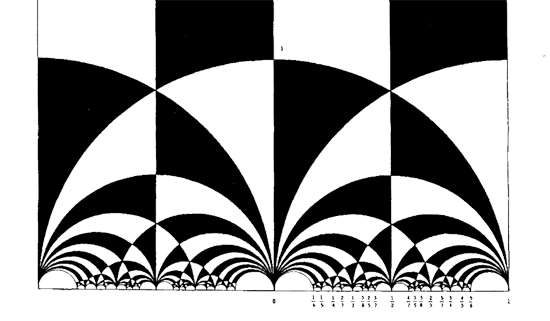So, all hyperbolic triangles we will encounter below are colored black or white. Now, take a Farey symbol and consider its associated special polygon in the hyperbolic plane. If we start with the Farey symbol

$$\xymatrix{\infty \ar@{-}_{(1)}[r] & 0 \ar@{-}_{\bullet}[r] & 1 \ar@{-}_{(1)}[r] & \infty}$$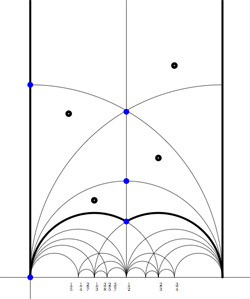we get the special polygonal region bounded by the thick edges, the vertical edges are identified as are the two bottom edges. Hence, this fundamental domain has 6 vertices (the 5 blue dots and the point at $i \infty$) and 8 hyperbolic triangles (4 colored black, indicated by a black dot, and 4 white ones).

Right, now let us associate a quiver to this triangulation (which embeds the quiver in the corresponding Riemann surface). The vertices of the triangulation are also the vertices of the quiver (so in our case we are going for a quiver with 6 vertices). Every hyperbolic edge in the triangulation gives one arrow in the quiver between the corresponding vertices. The orientation of the arrow is determined by the color of a triangle of which it is an edge : if the triangle is black, we run around its edges counter-clockwise and if the triangle is white we run over its edges clockwise (that is, the orientation of the arrow is independent of the choice of triangles to determine it). In our example, there is one arrows directed from the vertex at $i$ to the vertex at $0$, whether you use the black triangle on the left to determine the orientation or the white triangle on the right. If we do this for all edges in the triangulation we arrive at the quiver below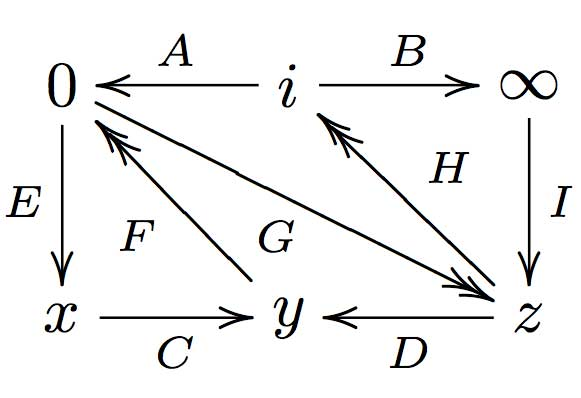where x,y and z are the three finite vertices on the $\frac{1}{2}$-axis from bottom to top and where I’ve used the physics-convention for double arrows, that is there are two F-arrows, two G-arrows and two H-arrows. Observe that the quiver is of Calabi-Yau type meaning that there are as much arrows coming into a vertex as there are arrows leaving the vertex.

Now that we have our quiver we determine the superpotential as follows. Fix an orientation on the Riemann surface (for example counter-clockwise) and sum over all black triangles the product of the edge-arrows counterclockwise MINUS sum over all white triangles
the product of the edge arrows counterclockwise. So, in our example we have the cubic superpotential

$IH’B+HAG+G’DF+FEC-BHI-H’G’A-GFD-CEF’$

From this we get the associated noncommutative algebra, which is the quotient of the path algebra of the above quiver modulo the following ‘commutativity relations’

$\begin{cases} GH &=G’H’ \\ IH’ &= IH \\ FE &= F’E \\ F’G’ &= FG \\ CF &= CF’ \\ EC &= GD \\ G’D &= EC \\ HA &= DF \\ DF’ &= H’A \\ AG &= BI \\ BI &= AG’ \end{cases}$

and morally this should be a Calabi-Yau algebra (( can someone who knows more about CYs verify this? )). This concludes the walk through of the procedure. Summarizing : to every Farey-symbol one associates a Calabi-Yau quiver and superpotential, possibly giving a Calabi-Yau algebra!

Yesterday, Ed Segal gave a talk at the Arts. His title “Superpotential algebras from 3-fold singularities” didnt look too promising to me. And sure enough it was all there again : stringtheory, D-branes, Calabi-Yaus, superpotentials, all the pseudo-physics babble that spreads virally among the youngest generation of algebraists and geometers.

Fortunately, his talk did contain a general ringtheoretic gem. After a bit of polishing up this gem, contained in his paper The A-infinity Deformation Theory of a Point and the Derived Categories of Local Calabi-Yaus, can be stated as follows.

Let $A$ be a $\mathbb{C}$-algebra and let $M = S_1 \oplus \ldots \oplus S_k$ be a finite dimensional semi-simple representation with distinct simple components. Let $\mathfrak{m}$ be the kernel of the algebra epimorphism $A \rightarrow S$ to the semi-simple algebra $S=End(M)$. Then, the $\mathfrak{m}$-adic completion of $A$ is Morita-equivalent to the completion of a quiver-algebra with relations. The nice thing is that both the quiver and relations come in a canonical way from the $A_{\infty}$-structure on the Ext-algebra $Ext^{\bullet}_A(M,M)$. More precisely, there is an isomorphism

$\hat{A}_{\mathfrak{m}} \simeq \frac{\hat{T}_S(Ext^1_A(M,M)^{\ast})}{(Im(HMC)^{\ast})}$

where the homotopy Maurer-Cartan map comes from the $A_{\infty}$ structure maps

$HMC = \oplus_i m_i~:~T_S(Ext_A^1(M,M)) \rightarrow Ext^2_A(M,M)$

and hence the defining relations of the completion are given by the image of the dual of this map.

For ages, Ive known this result in the trivial case of formally smooth algebras (where $Ext^2_A(M,M)=0$ and hence there are no relations to divide out) and where it is a consequence of a special case of the Cuntz-Quillen “tubular neighborhood” result. Completions of formally smooth algebras at semi-simples are Morita equivalent to completions of path algebras. This fact motivated all the local-quiver technology that was developed here in Antwerp over the last decade (see my book if you want to know the details).

Also for 3-dimensional Calabi-Yau algebras it states that the completions at semi-simples are Morita equivalent to completions of quotients of path algebras by the relations coming from a superpotential (aka a necklace) by taking partial noncommutative derivatives. Here the essential ingredient is that $Ext^2_A(M,M)^{\ast} \simeq Ext^1_A(M,M)$ in this case.

For any finite dimensional A-representation S we defined before a character $\chi(S)$ which is an linear functional on the noncommutative functions $\mathfrak{g}_A = A/[A,A]_{vect}$ and defined via

$\chi_a(S) = Tr(a | S)$ for all $a \in A$

We would like to have enough such characters to separate simples, that is we would like to have an embedding

$\mathbf{simp}~A \hookrightarrow \mathfrak{g}_A^*$

from the set of all finite dimensional simple A-representations $\mathbf{simp}~A$ into the linear dual of $\mathfrak{g}_A^*$. This is a consequence of the celebrated Artin-Procesi theorem.Michael Artin was the first person to approach representation theory via algebraic geometry and geometric invariant theory. In his 1969 classical paper “On Azumaya algebras and finite dimensional representations of rings” he introduced the affine scheme $\mathbf{rep}_n~A$ of all n-dimensional representations of A on which the group $GL_n$ acts via basechange, the orbits of which are exactly the isomorphism classes of representations. He went on to use the Hilbert criterium in invariant theory to prove that the closed orbits for this action are exactly the isomorphism classes of semi-simple -dimensional representations. Invariant theory tells us that there are enough invariant polynomials to separate closed orbits, so we would be done if the caracters would generate the ring of invariant polynmials, a statement first conjectured in this paper.Claudio Procesi was able to prove this conjecture in his 1976 paper “The invariant theory of $n \times n$ matrices” in which he reformulated the fundamental theorems on $GL_n$-invariants to show that the ring of invariant polynomials of m $n \times n$ matrices under simultaneous conjugation is generated by traces of words in the matrices (and even managed to limit the number of letters in the words required to $n^2+1$). Using the properties of the Reynolds operator in invariant theory it then follows that the same applies to the $GL_n$-action on the representation schemes $\mathbf{rep}_n~A$.

So, let us reformulate their result a bit. Assume the affine $\mathbb{C}$-algebra A is generated by the elements $a_1,\ldots,a_m$ then we define a necklace to be an equivalence class of words in the $a_i$, where two words are equivalent iff they are the same upto cyclic permutation of letters. For example $a_1a_2^2a_1a_3$ and $a_2a_1a_3a_1a_2$ determine the same necklace. Remark that traces of different words corresponding to the same necklace have the same value and that the noncommutative functions $\mathfrak{g}_A$ are spanned by necklaces.

The Artin-Procesi theorem then asserts that if S and T are non-isomorphic simple A-representations, then $\chi(S) \not= \chi(T)$ as elements of $\mathfrak{g}_A^*$ and even that they differ on a necklace in the generators of A of length at most $n^2+1$. Phrased differently, the array of characters of simples evaluated at necklaces is a substitute for the clasical character-table in finite group theory.

I have
been posting before on the necklace Lie algebra : on Travis
Schedler's extension of the Lie algebra structure to a Lie bialgebra
and its deformation and more recently
in connection with Michel Van den Bergh's double Poisson paper.
Yesterday, Victor Ginzburg and Travis Schedler posted their paper Moyal quantization of
necklace Lie algebras
on the arXiv in which they give a Moyal-type
construction of the Hopf algebra deformation of the necklace Lie
bialgebra found by Schedler last year.
It would be nice if
someone worked out a few examples of these constructions in full detail.
But as often in the case of (wild) quiver situation it is not clear what
an 'interesting' example might be. For the finite and tame case
we have a full classification by (extended) Dynkin diagrams so a natural
class of examples but it isn't clear how to find gems in the
complement.
One natural source of double quiver situations seems
to come from what I called the One Quiver of a
formally smooth algebra. This one quiver of group algebras of some
interesting arithemetical groups such as the modular group
$PSL_2(\mathbb{Z})$ and $SL_2(\mathbb{Z})$ were calculated before and
turned out to be consisting of one (resp. two) components which are the
double of the tame quiver $\tilde{A}_5$.
To obtain the double of
a wild quiver situation loook at the group $GL_2(\mathbb{Z}) = D_4 \bigstar_{D_2} D_6$. In a previous post
I thought to have calculated it, but lately I found that this was
incorrect. Even the version I computed last week still had some mistakes
as Raf
Bocklandt
discovered. But as of yesterday we are pretty certain that
the one quiver for $GL_2(\mathbb{Z})$ consists of two components. One of
these is the double quiver of an interesting wild quiver

$\xymatrix{& \vtx{} \ar@{=}[rr] \ar@{=}[dd] & & \vtx{} \ar@{=}[dd] \\ \vtx{} \ar@{=}[ur] \ar@{=}[rr] \ar@{=}[dd] & & \vtx{} \ar@{.}[ur] \ar@{.}[dd] \ar@{=}[dr] \\ & \vtx{} \ar@{.}[rr] \ar@{=}[dr] & & \vtx{} \\ \vtx{} \ar@{=}[rr] \ar@{.}[ur] & & \vtx{} \ar@{=}[ur]}$

where each double line indicates that there is an arrow in each
direction between the vertices. So, it is an interwoven pattern of one
big cycle of length 6 (reminiscent of the modular group case) with 4
cycles of length 5. Perhaps the associated necklace Lie (bi)algebra and
its deformation might be interesting to work out.
However, the
second component of the one quiver for $GL_2(\mathbb{Z})$ is _not_
symmetric.Maybe I will come back to the calculation of these quivers
later.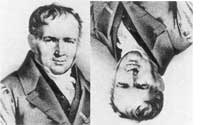This morning,
Michel Van den Bergh
posted an interesting paper on the arXiv
entitled Double
Poisson Algebras
. His main motivation was the construction of a
natural Poisson structure on quotient varieties of representations of
deformed multiplicative preprojective algebras (introduced by
Crawley-Boevey and Shaw in Multiplicative
preprojective algebras, middle convolution and the Deligne-Simpson
problem
) which he achieves by extending his double Poisson structure
on the path algebra of the quiver to the 'obvious' universal
localization, that is the one by inverting all $1+aa^{\star}$ for $a$ an
arrow and $a^{\star}$ its double (the one in the other direction).
For me the more interesting fact of this paper is that his double
bracket on the path algebra of a double quiver gives finer information
than the _necklace Lie algebra_ as defined in my (old) paper with Raf
Bocklandt Necklace
Lie algebras and noncommutative symplectic geometry
. I will
certainly come back to this later when I have more energy but just to
wet your appetite let me point out that Michel calls a _double bracket_
on an algebra $A$ a bilinear map
$\{ \{ -,- \} \}~:~A \times A \rightarrow A \otimes A$
which is a derivation in the _second_
argument (for the outer bimodulke structure on $A$) and satisfies
$\{ \{ a,b \} \} = – \{ \{ b,a \} \}^o$ with $~(u \otimes v)^0 = v \otimes u$
Given such a double bracket one can define an ordinary
bracket (using standard Hopf-algebra notation)
$\{ a,b \} = \sum \{ \{ a,b \} \}_{(1)} \{ \{ a,b \} \}_{(2)}$
which makes $A$ into
a Loday
algebra
and induces a Lie algebra structure on $A/[A,A]$. He then
goes on to define such a double bracket on the path algebra of a double
quiver in such a way that the associated Lie structure above is the
necklace Lie algebra.

The
previous post in this sequence was [moduli spaces]. Why did we spend
time explaining the connection of the quiver
$Q~:~\xymatrix{\vtx{} \ar[rr]^a & & \vtx{} \ar@(ur,dr)^x}$
to moduli spaces of vectorbundles on curves and moduli spaces of linear
control systems? At the start I said we would concentrate on its _double
quiver_ $\tilde{Q}~:~\xymatrix{\vtx{} \ar@/^/[rr]^a && \vtx{} \ar@(u,ur)^x \ar@(d,dr)_{x^*} \ar@/^/[ll]^{a^*}}$ Clearly,
this already gives away the answer : if the path algebra $C Q$
determines a (non-commutative) manifold $M$, then the path algebra $C \tilde{Q}$ determines the cotangent bundle of $M$. Recall that for a
commutative manifold $M$, the cotangent bundle is the vectorbundle
having at the point $p \in M$ as fiber the linear dual $(T_p M)^*$ of
the tangent space. So, why do we claim that $C \tilde{Q}$
corresponds to the cotangent bundle of $C Q$? Fix a dimension vector
$\alpha = (m,n)$ then the representation space
$\mathbf{rep}_{\alpha}~Q = M_{n \times m}(C) \oplus M_n(C)$ is just
an affine space so in its point the tangent space is the representation
space itself. To define its linear dual use the non-degeneracy of the
_trace pairings_ $M_{n \times m}(C) \times M_{m \times n}(C) \rightarrow C~:~(A,B) \mapsto tr(AB)$ $M_n(C) \times M_n(C) \rightarrow C~:~(C,D) \mapsto tr(CD)$ and therefore the linear dual
$\mathbf{rep}_{\alpha}~Q^* = M_{m \times n}(C) \oplus M_n(C)$ which is
the representation space $\mathbf{rep}_{\alpha}~Q^s$ of the quiver
$Q^s~:~\xymatrix{\vtx{} & & \vtx{} \ar[ll] \ar@(ur,dr)}$
and therefore we have that the cotangent bundle to the representation
space $\mathbf{rep}_{\alpha}~Q$ $T^* \mathbf{rep}_{\alpha}~Q = \mathbf{rep}_{\alpha}~\tilde{Q}$ Important for us will be that any
cotangent bundle has a natural _symplectic structure_. For a good
introduction to this see the [course notes] “Symplectic geometry and
quivers” by [Geert Van de Weyer]. As a consequence $C \tilde{Q}$
can be viewed as a non-commutative symplectic manifold with the
symplectic structure determined by the non-commutative 2-form
$\omega = da^* da + dx^* dx$ but before we can define all this we
will have to recall some facts on non-commutative differential forms.
Maybe [next time]. For the impatient : have a look at the paper by
Victor Ginzburg [Non-commutative Symplectic Geometry, Quiver varieties,
and Operads] or my paper with Raf Bocklandt [Necklace Lie algebras
and noncommutative symplectic geometry]. Now that we have a
cotangent bundle of $C Q$ is there also a _tangent bundle_ and does it
again correspond to a new quiver? Well yes, here it is
$\xymatrix{\vtx{} \ar@/^/[rr]^{a+da} \ar@/_/[rr]_{a-da} & & \vtx{} \ar@(u,ur)^{x+dx} \ar@(d,dr)_{x-dx}}$ and the labeling of the
arrows may help you to work through some sections of the Cuntz-Quillen
paper…

: http://www.neverendingbooks.org/index.php?p=39
: http://www.win.ua.ac.be/~gvdwey/lectures/symplectic_moment.pdf
: http://www.win.ua.ac.be/~gvdwey/
: http://www.neverendingbooks.org/index.php?p=41
: http://www.arxiv.org/abs/math.QA/0005165
: http://www.arxiv.org/abs/math.AG/0010030

Now that the preparation for my undergraduate courses in the first semester is more or less finished, I can begin to think about the courses I’ll give this year in the master class
non-commutative geometry. For a change I’d like to introduce the main ideas and concepts by a very concrete example : Ginzburg’s coadjoint-orbit result for the Calogero-Moser space and its
relation to the classification of one-sided ideals in the first Weyl algebra. Not only will this example give me the opportunity to say things about formally smooth algebras, non-commutative
differential forms and even non-commutative symplectic geometry, but it also involves what some people prefer to call _non-commutative algebraic geometry_ (that is the study of graded Noetherian
rings having excellent homological properties) via the projective space associated to the homogenized Weyl algebra. Besides, I have some affinity with this example.

A long time ago I introduced
the moduli spaces for one-sided ideals in the Weyl algebra in Moduli spaces for right ideals of the Weyl algebra and when I was printing a _very_ preliminary version of Ginzburg’s paper
Non-commutative Symplectic Geometry, Quiver varieties, and Operads (probably because he send a preview to Yuri Berest and I was in contact with him at the time about the moduli spaces) the
idea hit me at the printer that the right way to look at the propblem was to consider the quiver

$\xymatrix{\vtx{} \ar@/^/[rr]^a & & \vtx{} \ar@(u,ur)^x \ar@(d,dr)_y \ar@/^/[ll]^b}$

which eventually led to my paper together with Raf Bocklandt Necklace Lie algebras and noncommutative symplectic geometry.

Apart from this papers I would like to explain the following
papers by illustrating them on the above example : Michail Kapranov Noncommutative geometry based on commutator expansions Maxim Kontsevich and Alex Rosenberg Noncommutative smooth
spaces
Yuri Berest and George Wilson Automorphisms and Ideals of the Weyl Algebra Yuri Berest and George Wilson Ideal Classes of the Weyl Algebra and Noncommutative Projective
Geometry
Travis Schedler A Hopf algebra quantizing a necklace Lie algebra canonically associated to a quiver and of course the seminal paper by Joachim Cuntz and Daniel Quillen on
quasi-free algebras and their non-commutative differential forms which, unfortunately, in not available online.

I plan to write a series of posts here on all this material but I will be very